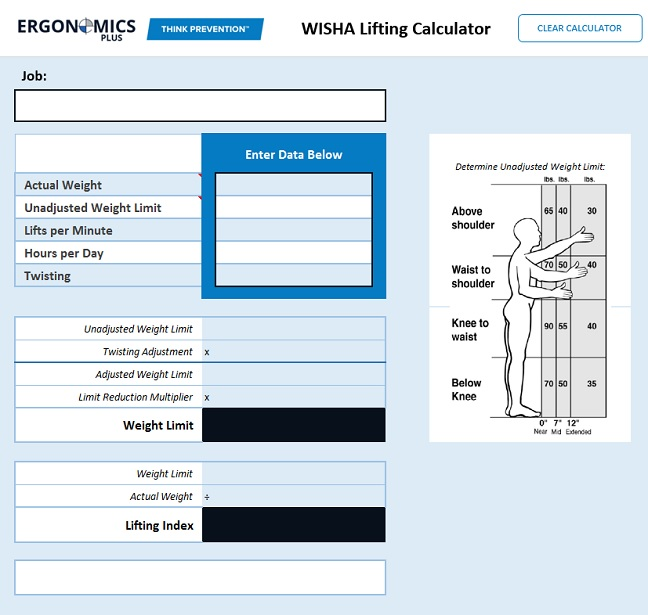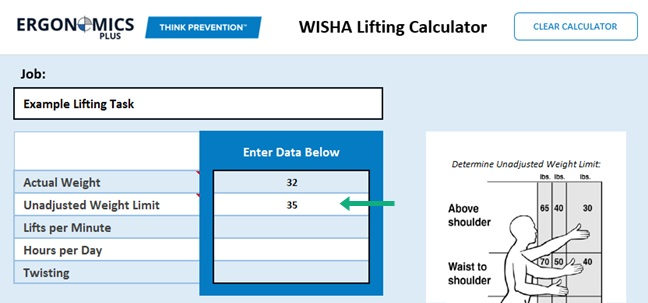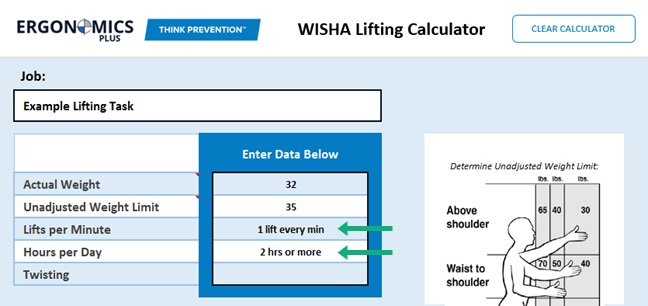# Lifting Calculator

Learn how to use the WISHA Lifting Calculator to evaluate manual material handling tasks.### Introduction to the WISHA Lifting Calculator

The WISHA Lifting Calculator is a great ergonomic evaluation tool for manual material handling tasks.

Developed by the Washington State Department of Labor and Industries, this lifting calculator is very simple in design and application.

This ergonomic assessment tool is an adaptation of the NIOSH Lifting Equation (1,2), which is based on scientific research on the primary causes of work-related back injuries. This calculator can be used to perform simple ergonomic risk assessments on a wide variety of manual lifting and lowering tasks, and can be also used as a screening tool to identify lifting tasks which should be analyzed further using the more comprehensive NIOSH Lifting Equation.

### Data Collection

To use the WISHA Lifting Calculator, you will first need to collect the data needed to perform the evaluation. This information can be obtained by observing the job being performed, interviewing workers, and reviewing productivity and other work records.

You will need the following information for each lifting or lowering task evaluated:

• Weight of objects the employee lifts.
• Location or posture when employee performs lift.
• Frequency of lifting – number of times employee performs lift.
• Duration of lifting – number of hours per day spent lifting.

This information will then be entered into the WISHA Lifting Calculator to determine the Weight Limit and Lifting Index for the lift.Jobs that require a wide variety of lifting and lowering tasks are a little harder to evaluate. If you are evaluating a multi-task job, I would suggest that you keep it simple by analyzing lifting requirements in two or three ways.

For example:

1. Analyze the worst case data by using the heaviest objects lifted from the most awkward positions. Use the frequency for these lifts only, not the total number of lifts. It’s not accurate to use the heaviest weight lifted with the frequency for all lifting, if there is a wide variety of lifting required.
2. Analyze the most commonly performed lifts.
3. Perform the analysis by using the average weight and position, using the frequency and duration for all of the lifting done in a typical workday.

### Example: Using the WISHA Lifting Calculator

Step 1: Find the actual weight of objects the employee lifts. For multi-task jobs with a variety of lifting and lowering, you can use the heaviest object lifted and/or an average weight. In this example, the average weight lifted is 32 lbs.Step 2: Determine the Unadjusted Weight Limit.  Where are the employee’s hands when they begin to lift or lower the object? Note that spot on the calculator diagram. The number in that box is the Unadjusted Weight Limit in pounds. To determine the horizontal reach, measure the midpoint between the hands from the midpoint between the ankles.Unadjusted Weight Limit = 35 lbs.Step 3: Find the Limit Reduction Modifier.

Find out how many times the employee lifts per minute and the total number of hours per day spent lifting.  Enter this information into the calculator and the Limit Reduction Modifier will be calculated for you.

In this example, the employee lifts once per minute for two hours or more.Select the twisting adjustment from the dropdown menu. If the employee is required by the job to twist more than 45 degrees while lifting, the Unadjusted Weight Limit is reduced by multiplying by 0.85.Step 5: Calculate and Review Results

After entering the information in the calculator input boxes, the Weight Limit and Lifting Index will be calculated for you.

Compare the Weight Limit (26.25 lbs.) with the Actual Weight lifted (32 lbs.) from Step 1.  If the Actual Weight lifted is greater than the Weight Limit calculated, then the lifting is a WMSD hazard and must be reduced below the hazard level or to the degree technologically and economically feasible.

In this example, the actual Weight of 32 lbs. is greater than the Weight Limit of 26.25 lbs. Therefore, this lifting task is considered a hazard and control measures should be implemented if possible.Lifting Index

The Lifting Index (LI) provides a relative estimate of the level of physical demands and stress associated with a particular manual lifting or lowering task. The estimate of the level of physical stress is defined by the relationship or ratio of the weight of the Actual Weight and the Weight Limit. The Lifting Index is defined by the following equation:

Actual Weight ÷ Weight Limit = Lifting Index

In our example lifting task above, we can calculate the Lifting Index as follows:

32 lbs. ÷ 26.25 lbs. = 1.22 Lifting Index = 1.22

The higher the Lifting Index, the smaller the percentage of workers capable of safely performing the lifting and lowering job demands over time and the greater the injury risk. The ergonomic goal should be to design all lifting jobs to achieve a Lifting Index  of 1.0 or less.

The Lifting Index great tool to compare the relative risk of multiple job tasks and prioritize ergonomic improvement efforts. For example, several high risk jobs could be ranked in order according to the Lifting Index and a control strategy could be developed according to the rank ordering.